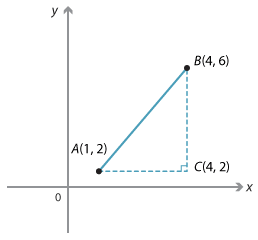### General case

The examples above considered the special cases when the line interval AB is either horizontal or vertical. Pythagoras' theorem is used to calculate the distance between two points when the line interval between them is neither vertical nor horizontal.Detailed description

The distance between the points A(1, 2) and B(4, 6) is calculated below.

AC = 4 − 1 = 3 and BC = 6 − 2 = 4.

By Pythagoras' theorem:

\begin{align}AB^2  &= 3^2 + 4^2 = 25\\\\ AB &= \sqrt{25}\\\\ \text{And so}, AB &= 5.\end{align}